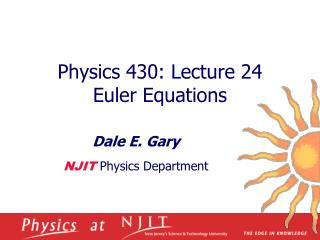DownloadDownload PresentationPhysics 430: Lecture 24 Euler Equations

# Physics 430: Lecture 24 Euler Equations

Télécharger la présentation## Physics 430: Lecture 24 Euler Equations

- - - - - - - - - - - - - - - - - - - - - - - - - - - E N D - - - - - - - - - - - - - - - - - - - - - - - - - - -
##### Presentation Transcript

1. Physics 430: Lecture 24 Euler Equations Dale E. Gary NJIT Physics Department

2. 10.7 Euler Equations • We now know that all objects have three principal axes, and that we can always write the inertia tensor as a diagonal matrix relative to these axes, so that the angular momentum vector can be written L = (l1w1, l2w2, l3w3). • In the accelerating (rotating) frame of the body (we will call it the body frame), the body is stationary with respect to the rotation. The inertial (non-rotating) frame is traditionally called the space frame. • We can use coordinates x, y, z as the coordinates in the space frame, and e1, e2, e3 as unit vectors in the directions of the principle axes in the body frame. • Recall that the time derivative of the angular momentum is the torque: • We can transform to the body frame by adding the usual w×L: • This leads us to Euler’s equation:

3. Euler Equations-2 • Since L = (l1w1, l2w2, l3w3), we have . • Therefore, the Euler vector equation can be written as three equations: • These equations give the evolution of w in the frame of the body, but in general they are difficult to use because they require knowledge of the components G1, G2 and G3 of the applied torque, which in the rotating frame are complicated. • They are useful, however, in cases where the torque is zero, and that is the case we will discuss further. • Meanwhile, for the example of the spinning top we have the case that the torque is always perpendicular to e3, so G3 is zero. Also, because of the symmetry of the top, l1 = l2. The third equation above then says • This means the component of w along the symmetry axis is constant.

4. 10.8 Euler Equations with Zero Torque • In the case of zero torque, Euler’s Equations become: • We will first consider these equations when the three principal moments are all different, and then for the case, as for the spinning top, that l1 = l2. • One thing we can see immediately is that if the rotation axis starts out pointing along one of the principal axes (e.g. w = wi), it will stay in that direction. That is, two of the equations will be zero, and the third (ith) equation says w = constant. • Conversely, if the rotation axis is NOT along one of the principle axes, its direction will not be constant. To see this, note that at least two of the components of w must be non-zero, which means at least one component of must be non-zero. • So, rotation about a principal axis is the only way to have a constant rotation axis. An interesting question is whether such a rotation is stable—that is, if we slightly perturb the rotation will it grow with time?

5. Stability Against Perturbation • To answer this, let’s assume we initially have rotation about the 3 axis, so that w1 = w2 = 0. If we give the body a small tap, so that both of these become non-zero, but small, then the third Euler equation says will be “doubly small”. If we take it to be constant, then we have only the first two equations where the quantities in square brackets are (approximately) constant. • As usual, we can take the time derivative of the first and substitute into the second to get • You can recognize this immediately as simple harmonic motion in w1 with the oscillation frequency • But note that the radical could be negative, which means w is imaginary. Stable if l3 > l1 and l2 or if l3 < l1 and l2

6. stable unstable stable Stability Against Perturbation-2 • We therefore have the interesting situation that if you spin an object about either its longest or shortest axes, it will be stable, but around its intermediate axis, it is unstable. Stability of a Top • Now let’s consider the case, as for the spinning top, that l1 = l2. In this case, we have • Then we can write the other two Euler equations in terms of a constant frequency Wb: h = w1 + iw2

7. e3 (fixed) e3 w w L L(fixed) Stability of a Top h = w1 + iw2 • This has solution: • Let’s take initial conditions w1 = wo and w2 = 0 at t = 0. Then ho = wo, and when we put in the real and imaginary parts of h, the complete solution is: with wo and w3 both constant. • The relationship between w1 and w2 is that of a rotating (precessing) vector component, precessing with frequency Wb. Therefore, as seen from the body frame (the one rotating with angular frequency w3 in the direction e3), the vector w moves steadily around a cone, called the body cone., centered on the direction e3. • Meanwhile, the angular momentum L is give by • In the space frame, w precesses about L. at a different frequency, Wb = L/l1. • This is called free precession, and does not require a torque. Wb Ws body frame space frame